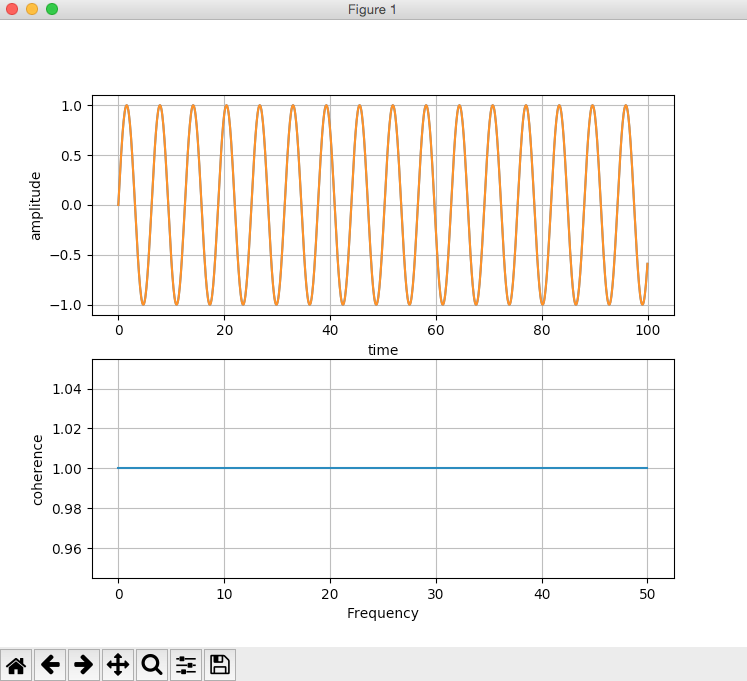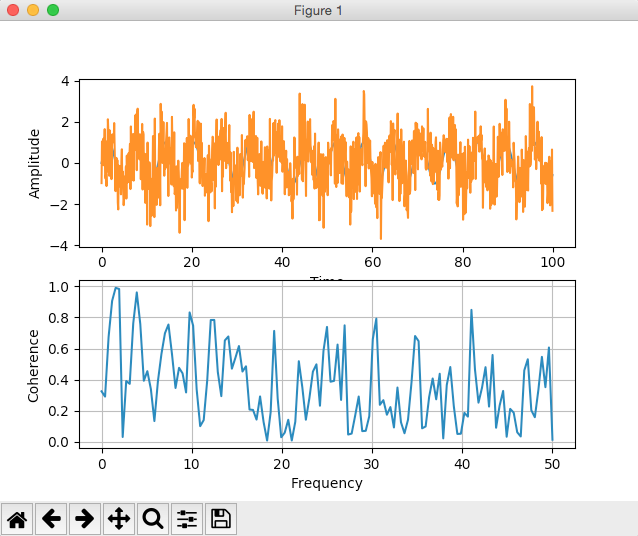# Coherence - Plotting The Coherence Between Two Signals Using Python And Matplotlib

Overview:

Before going to plot coherence between two signals let us do some recap required for definitions in Digital Signal Processing.

Signals represent information and systems process them. Signals carry information, energy and so on.  A signal is a function of some variable, which is described over time, space, or some other variable.

## Examples of Signals:

• Sunrise, sunset and the sunlight are signals received by birds, animals and human beings and they take some action like waking up in the morning, eating and sleeping.

• A car turning on a curve flashing the blinkers either on the right or left hand side

• Varying of voltage over a time periodCourtesy: Andrew

## Coherence in Digital Signal Processing:

• Coherence is a measure used for comparing the relation between two signals. It is based on the correlation between two signals.

• Coherence measures the normalized correlation between two power spectra.  A power spectra tells how much of the power energy is contained in the frequency components of the signal.

Coherence is mathematically given by

Cxy(f) = |Gxy(f)|^2/Gxx(f)Gyy(f)

Gxy(f) - Cross Spectral Density of the signals x and y

Gxx(f)- Power Spectral density of signal x

Gyy(f)- Power Spectral density of y

• If coherence is 1 both the signals are fully in coherence that is if signal one is the input and signal two is the output, signal one can be characterized fully using signal 2. This is the ideal characteristic of a linear system.
• If coherence is less than 1 both the signals are in coherence + some noise.
• If coherence is zero the signals one and two are not related.

## Plotting Coherence between two signals using Python and Matplotlib:

Pyplot module of Matplotlib library provides cohere() method, which calculates the coherence and the frequencies of the coherence vector.

## Example1: (Where Coherence =1,between the two signals)

The following python example creates two identical sine waves using matplotlib and calculates the coherence between them. Since both the signals are identical the coherence between the two signals is 1. The coherence value 1 is visualized as a straight line along the X-axis of the plot.

 # import numpy and pyplot modules import numpy as np import matplotlib.pyplot as plot   # Create sine wave1 time        = np.arange(0, 100, 0.1) sinewave1   = np.sin(time)   # Create sine wave2 as replica of sine wave1 time1        = np.arange(0, 100, 0.1) sinewave2    = np.sin(time1)   # Plot the sine waves - subplot 1 plot.title('Two sine waves with coherence as 1') plot.subplot(211) plot.grid(True, which='both') plot.xlabel('time') plot.ylabel('amplitude') plot.plot(time, sinewave1, time1, sinewave2)   # Plot the coherence - subplot 2 plot.subplot(212) coh, f = plot.cohere(sinewave1, sinewave2, 256, 1./.01) print("Coherence between two signals:") print(coh) print("Frequncies of the coherence vector:") print(f) plot.ylabel('coherence') plot.show()

## Output1: (Where Coherence =1,between the two signals):Coherence between two signals: [ 1.  1.  1.  1.  1.  1.  1.  1.  1.  1.  1.  1.  1.  1.  1.  1.  1.  1.   1.  1.  1.  1.  1.  1.  1.  1.  1.  1.  1.  1.  1.  1.  1.  1.  1.  1.   1.  1.  1.  1.  1.  1.  1.  1.  1.  1.  1.  1.  1.  1.  1.  1.  1.  1.   1.  1.  1.  1.  1.  1.  1.  1.  1.  1.  1.  1.  1.  1.  1.  1.  1.  1.   1.  1.  1.  1.  1.  1.  1.  1.  1.  1.  1.  1.  1.  1.  1.  1.  1.  1.   1.  1.  1.  1.  1.  1.  1.  1.  1.  1.  1.  1.  1.  1.  1.  1.  1.  1.   1.  1.  1.  1.  1.  1.  1.  1.  1.  1.  1.  1.  1.  1.  1.  1.  1.  1.   1.  1.  1.] Frequencies of the coherence vector: [  0.         0.390625   0.78125    1.171875   1.5625     1.953125    2.34375    2.734375   3.125      3.515625   3.90625    4.296875   4.6875    5.078125   5.46875    5.859375   6.25       6.640625   7.03125    7.421875   7.8125     8.203125   8.59375    8.984375   9.375      9.765625   10.15625   10.546875  10.9375    11.328125  11.71875   12.109375  12.5   12.890625  13.28125   13.671875  14.0625    14.453125  14.84375   15.234375  15.625     16.015625  16.40625   16.796875  17.1875    17.578125   17.96875   18.359375  18.75      19.140625  19.53125   19.921875  20.3125   20.703125  21.09375   21.484375  21.875     22.265625  22.65625   23.046875  23.4375    23.828125  24.21875   24.609375  25.        25.390625   25.78125   26.171875  26.5625    26.953125  27.34375   27.734375  28.125   28.515625  28.90625   29.296875  29.6875    30.078125  30.46875   30.859375  31.25      31.640625  32.03125   32.421875  32.8125    33.203125   33.59375   33.984375  34.375     34.765625  35.15625   35.546875  35.9375   36.328125  36.71875   37.109375  37.5       37.890625  38.28125   38.671875  39.0625    39.453125  39.84375   40.234375  40.625     41.015625   41.40625   41.796875  42.1875    42.578125  42.96875   43.359375  43.75   44.140625  44.53125   44.921875  45.3125    45.703125  46.09375   46.484375  46.875     47.265625  47.65625   48.046875  48.4375    48.828125   49.21875   49.609375  50.      ]

## Example2: (Where Coherence < 1,between the two signals):

 #import numpy and pyplot import numpy as np import matplotlib.pyplot as plot   # Get x values of the sine wave one time        = np.arange(0, 100, 0.1);   # Create sine wave one sinewave1   = np.sin(time)   # Get x values of the sine wave two time1        = np.arange(0, 100, 0.1);   # Create sine wave two sinewave2    = np.sin(time1) + np.random.randn(len(time1)) # add white noise to the signal   # Plot the sine waves plot.subplot(211) plot.xlabel("Time") plot.ylabel("Amplitude") plot.plot(time, sinewave1, time1, sinewave2)   plot.subplot(212) coh, f = plot.cohere(sinewave1, sinewave2, 256, 1./.01)   print("Coherence between the sine wave one and sine wave two:") print(coh)   print("Frequency vector:") print(f)   plot.xlabel('Frequency') plot.ylabel('Coherence') plot.show()

## Output2: (Where Coherence < 1,between the two signals):

• In example2 we have introduced white noise.

• The coherence values between the signal1 and signal2 are given here. The coherence values are less than one but greater than zero, which means the two signals – sign wave1 and sign wave2 are coherent, but not fully. The noise present in signal2 prevents us describe the signal1 fully in terms of signal2.Coherence between the sine wave one and sine wave two: [ 0.32468261  0.29048112  0.6742881   0.90664218  0.99080677  0.98326528   0.03054029  0.39089757  0.37261148  0.76272111  0.96162723  0.75703402   0.39236145  0.45448182  0.33928063  0.133061    0.38922675  0.56463312   0.69878137  0.75483307  0.56327037  0.34606935  0.47585059  0.44014512   0.31727541  0.83267451  0.74711271  0.33736817  0.10024564  0.1394823   0.39596828  0.78368179  0.78405942  0.44949323  0.29349927  0.65211104   0.67787445  0.47020952  0.54027687  0.61634338  0.45184892  0.48577513   0.20729735  0.20482254  0.14241289  0.29173771  0.12684244  0.00758156   0.18933596  0.71362819  0.27851464  0.02872453  0.05843246  0.14154921   0.00777536  0.12888692  0.51855754  0.34939773  0.1416513   0.28053583   0.45158895  0.49850282  0.23170471  0.58504021  0.73921409  0.38627158   0.3918108   0.62565787  0.26903731  0.7495451   0.04623494  0.05255391   0.16883985  0.29124716  0.06835     0.07024807  0.16421559  0.66011261   0.79270229  0.23755552  0.2678224   0.1737412   0.22326423  0.09187296   0.349182    0.1253156   0.05452957  0.14229612  0.38483263  0.68075285   0.64764936  0.08630145  0.09837388  0.28872958  0.40759757  0.27310724   0.43783911  0.02144801  0.36593274  0.48107479  0.22854087  0.05025897   0.05140727  0.18605392  0.16155378  0.84900671  0.46646439  0.25157826   0.34727315  0.4801423   0.2262376   0.5587727   0.09000678  0.23270016   0.32704345  0.03202457  0.21167854  0.18721209  0.0609601   0.033863   0.45909516  0.53117697  0.20262965  0.15782563  0.33278989  0.54651145   0.35119042  0.60685768  0.01307827]

 Frequency vector: [  0.         0.390625   0.78125    1.171875   1.5625     1.953125    2.34375    2.734375   3.125      3.515625   3.90625    4.296875   4.6875    5.078125   5.46875    5.859375   6.25       6.640625   7.03125    7.421875   7.8125     8.203125   8.59375    8.984375   9.375      9.765625   10.15625   10.546875  10.9375    11.328125  11.71875   12.109375  12.5   12.890625  13.28125   13.671875  14.0625    14.453125  14.84375   15.234375  15.625     16.015625  16.40625   16.796875  17.1875    17.578125   17.96875   18.359375  18.75      19.140625  19.53125   19.921875  20.3125   20.703125  21.09375   21.484375  21.875     22.265625  22.65625   23.046875  23.4375    23.828125  24.21875   24.609375  25.        25.390625   25.78125   26.171875  26.5625    26.953125  27.34375   27.734375  28.125   28.515625  28.90625   29.296875  29.6875    30.078125  30.46875   30.859375  31.25      31.640625  32.03125   32.421875  32.8125    33.203125   33.59375   33.984375  34.375     34.765625  35.15625   35.546875  35.9375   36.328125  36.71875   37.109375  37.5       37.890625  38.28125   38.671875  39.0625    39.453125  39.84375   40.234375  40.625     41.015625   41.40625   41.796875  42.1875    42.578125  42.96875   43.359375  43.75   44.140625  44.53125   44.921875  45.3125    45.703125  46.09375   46.484375  46.875     47.265625  47.65625   48.046875  48.4375    48.828125   49.21875   49.609375  50.      ]